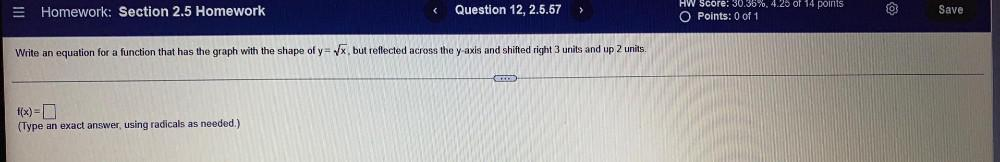Question:

# Write an equation for a function that has the graph with theWrite an equation for a function that has the graph with the shape of y=√x, but reflected across the y-axis and shifted right 3 units and up 2 units. f(x) =? (Type an exact answer, using radicals as needed.)